Goseeko blog# What is integration?￼

## Introduction (Integration)

Integration is the reverse process of differentiation. It is also called anti-differentiation.

Integral calculus has its own application in economics, Engineering, Physics, Chemistry, business, commerce, etc.

The integral of a function is denoted by the sign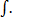Let the function is y = f(x),

Its derivative is-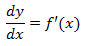Then

Where c is the arbitrary constant.

For example,

A function,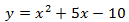Then, its derivative-

Or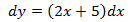Then-

Here c is an arbitrary constant.

## Methods of integration

Simple integration-

1.     When the function is an algebraic function-

Some standard results-

1. If dy = f’(x) dx, then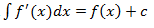2. If dy = a [f’(x) dx] where a is constant, then-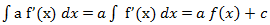3.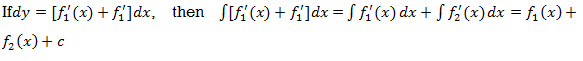The integration of x^n will be as follows-

Example: Find the integral of-

Sol:

We know that-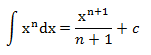hence,

Example: Find the integral

Sol.

We know that-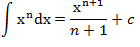then

## Integration by substitution

Example: Evaluate the following integral-

Sol:

Let us suppose,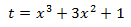or

therefore, Substituting –

## Logarithmic function

Example: Evaluate the following integral-

Sol:

Let us suppose-

## Integration of exponential function

Example: Evaluate-

Sol:

Let

Now substituting-

## Method for product of two functions

Suppose we have two function say- f(x) and g(x), then

The integral of product of these two functions is-

Note-

We chose the first function as method of ILATE-

Which is-

I – Inverse trigonometric function

L – Log function

A – Algebraic function

T- Trigonometric function

E- Exponential function

Example: Evaluate-

Sol:

Here according to ILATE,

First function = log x

Second function =x^n

We know that-

Then-

On solving, we get-

Interested in learning about similar topics? Here are a few hand-picked blogs for you!